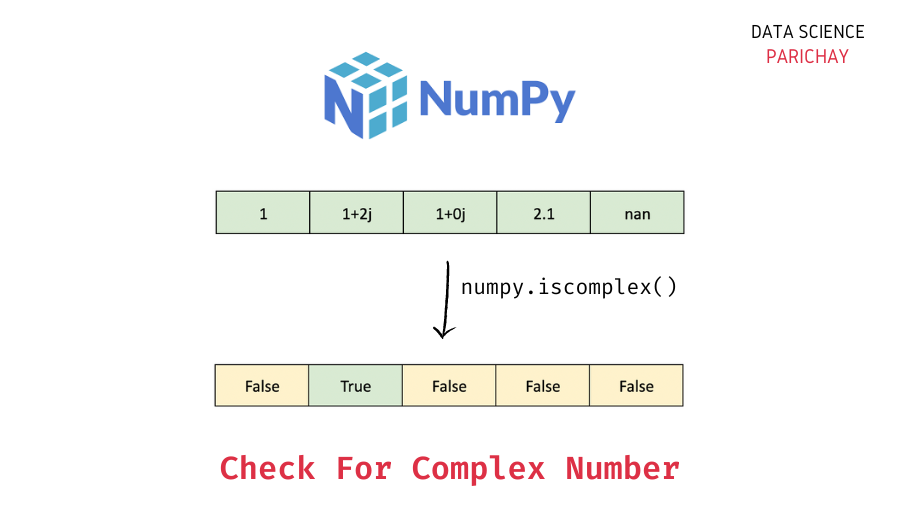# Numpy – Check If a Number is Complex

In this tutorial, we will look at how to check if a number in a Numpy array is complex or not with the help of some examples. Complex numbers in mathematics are numbers that have an imaginary part, for example, `1 + 2j`.

## How to test for a complex number in a Numpy array?

You can use the `numpy.iscomplex()` function to check (element-wise) if values in a Numpy array are complex or not. The following is the syntax –

```# test for complex numbers - pass scaler value or numpy array
np.iscomplex(a)```

The `numpy.iscomplex()` function essentially tests (element-wise) whether the number has a non-zero imaginary part or not. It returns a boolean array if you pass an array and if you pass a scaler value, it returns a boolean value.

## Examples

Let’s now look at some examples of using the above function to test for complex numbers.

### Example 1 – Check if a number is complex or not using `numpy.iscomplex()`

First, let’s pass scaler values to the `numpy.iscomplex()` function.

Let’s apply the `numpy.iscomplex()` function on three scaler values – a real number, a complex number with a non-zero imaginary part, and a complex number with the imaginary part as 0.

```import numpy as np

# check if complex
print(np.iscomplex(14))
print(np.iscomplex(2 + 4j))
print(np.iscomplex(2 + 0j))```

Output:

```False
True
False```

We get `False` as the output for the real number 14 and the complex number having 0 as its imaginary part. And we get `True` as the output for the complex number with a non-zero imaginary part.

📚 Data Science Programs By Skill Level

Introductory

Intermediate ⭐⭐⭐

🔎 Find Data Science Programs 👨‍💻 111,889 already enrolled

Disclaimer: Data Science Parichay is reader supported. When you purchase a course through a link on this site, we may earn a small commission at no additional cost to you. Earned commissions help support this website and its team of writers.

Note that here, even though the value `2 + 0j` is of `complex` type, the `numpy.iscomplex()` function returns it as not a complex value (since its imaginary part is 0 which essentially makes it a real value).

If, on the other hand, you want to check whether the value is of `complex` type, use the `numpy.iscomplexobj()` function.

```# check if complex type object
print(np.iscomplexobj(14))
print(np.iscomplexobj(2 + 4j))
print(np.iscomplexobj(2 + 0j))```

Output:

```False
True
True```

We get `False` as the output for the real number 14 and `True` as the output for both the complex type values (irrespective of whether the imaginary part is 0 or not).

### Example 2 – Element-wise check for complex number in a Numpy array using `numpy.iscomplex()`

If you apply the `numpy.iscomplex()` function on an array, it will return a boolean array containing `True` for the values that are complex and `False` for the other values.

Let’s create a 1-D array and apply the `numpy.iscomplex()` function to it.

```# create a numpy array
ar = np.array([1, 2+3j, 2+0j, 4, 5.7, np.nan, np.inf, 1j, 0+0j])
# element-wise check for complex value in ar
np.iscomplex(ar)```

Output:

`array([False,  True, False, False, False, False, False,  True, False])`

We get a boolean array as an output. You can see that in the boolean array we get `True` for only the values that test as a complex number in the original array.

## Summary

In this tutorial, we looked at how we can use the `numpy.iscomplex()` function to check if a number is complex or not in a Numpy array. Keep in mind that this function checks whether the imaginary part of the number is non-zero or not. If you want to check whether the number is of `complex` type, use the `numpy.iscomplexobj()` function instead.

You might also be interested in –

•